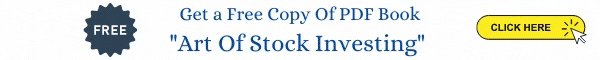What is the NAV?

A mutual fund is a common investment vehicle where the assets of the fund belong directly to the investors. Investors subscriptions are accounted for by the fund not as liabilities or deposits but as Unit Capital. On the other hand, the investments made on behalf of the investors are reflected on the asset side and are the main constituent of the mutual funds schemes balance sheet. There are, however, liabilities of a mainly short-term nature that may be part of the balance sheet. The funds total assets are therefore defined as the assets minus the liabilities. As there are many investors in the fund, it is common practice for mutual funds to compute the share of each investor on the basis of the value of Net Assets Per Unit, commonly known as the Net Asset Value (NAV).

NAV = Net Assets of the Scheme/Number of units outstanding
Net assets of the scheme = Market value of investments + receivables + other accrued income +other assets-accrued expenses-other payables-other liabilities.